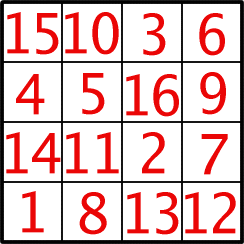#### You may also like### Exploring Wild & Wonderful Number Patterns

EWWNP means Exploring Wild and Wonderful Number Patterns Created by Yourself! Investigate what happens if we create number patterns using some simple rules.### Sending Cards

This challenge asks you to investigate the total number of cards that would be sent if four children send one to all three others. How many would be sent if there were five children? Six?### Dice and Spinner Numbers

If you had any number of ordinary dice, what are the possible ways of making their totals 6? What would the product of the dice be each time?

# Magic Constants

##### Age 7 to 11Challenge Level

This is a 4 x 4 Magic Square made from the numbers 1 to 16.In a Magic Square all the rows, columns and diagonals add to the same number. This number is called the 'Magic Constant'.

1/What is the Magic Constant of this Magic Square?

This particular square is especially 'magic' as some 2 x 2 squares within it also add to that number.

2/How many of these squares can you find?

3/What happens to the Magic Constant if you add 2 to each number in the square?

4/What happens if you double each number?

5/Can you make a square in which the Magic Constant is 17?

How did you do it?

6/Can you make a square in which the Magic Constant is 38?

How did you do it?

7/What other numbers under 100 can you make into the Magic Constant by changing all the numbers in the square in the same way?

8/Can some be made in more than one way?

9/Are there some numbers you really cannot make?